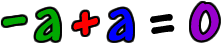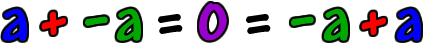Let's look at a number...  5...  Because this is my favorite number!What number can we add to 5 to get 0 (which is the additive identity) as the answer?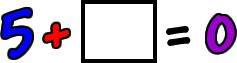You should be thinking about a negative number.  In fact, you should be thinking about a number that is the opposite of 5!  -5!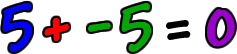So, the additive inverse of 5 is -5!

Let's go the other way...

What number can we add to -5 to get 0 as the answer?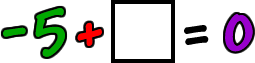What's the opposite of -5?  5!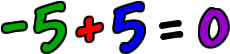So, the additive inverse of -5 is 5!

Here's the official property:

 The additive inverse of a number,isso that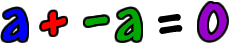and